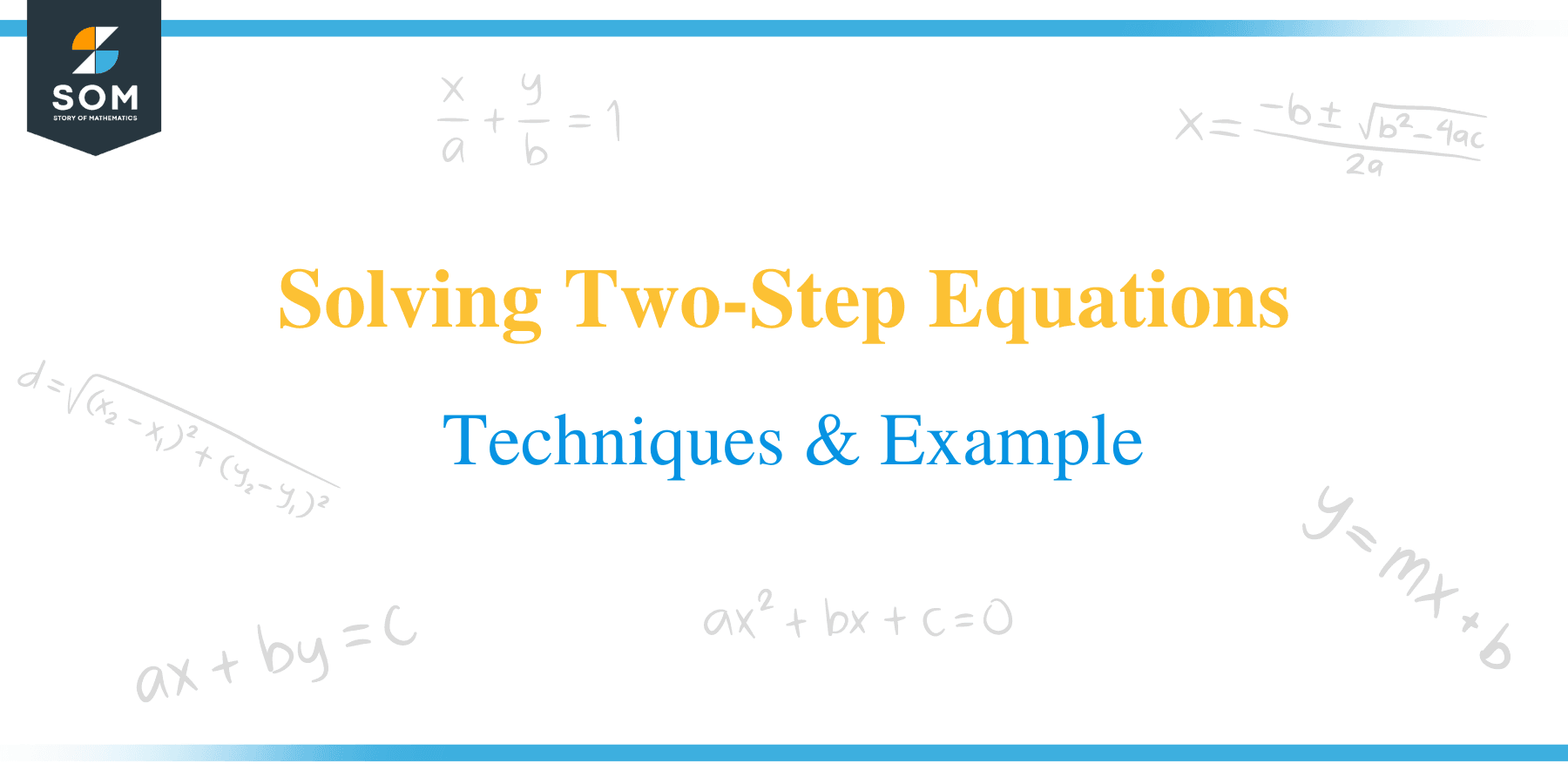# Solving Two-Step Equations – Techniques & Examples

##What is a Two-step Equation?

It is probably undebatable that a two-step equation is as easy as ABC. As the name suggests, a two-step equation is an algebraic equation that requires only two steps to be completely solved.

The equation is already solved when the value of the variable is found. In this article, we will take you step by step in solving two-step equations to make you familiar and proficient with the process.

Generally, when solving an equation, we apply the Law of Equations, which states that whatever is to be performed on the right-hand side (RHS) of an equation should also be done to the left-hand side (LHS) of the equation so that the equation can remain balanced.

A two-step equation has been solved if a variable, usually represented by an alphabetical letter, is isolated on either the equation’s left or right side. The number is located on the opposite side.

## How to Solve Two-step Equations?

Solving a two-step equation involves working backward concerning the order of operations (PEMDAS). In this case, multiplication and division are preceded by addition and subtraction.

Tips for Solving Two-step equations include:

• Always apply addition or subtraction to remove a constant.
• Apply multiplication or division to remove any coefficient from a variable.Example 1

Solve the two-step equation y:

3y – 2 = 13

Solution

Add 2 to both sides of the equation and divide by 3.

3y – 2 + 2 = 13 + 2

3y = 15

3y/3 = 15/3

y = 5

Example 2

Solve the two-step equation for z.

2z +15 = −3z

Solution

Subtract 2z from both sides of the equation and divide by -5.

2z – 2z + 15 = -3z – 2z

15 = -5z

15/-5 = -5z/-5

z = 3

Example 3

Solve the two-step equation for x

(x/5) -6 = -8

Solution

Add both 6 to both sides of the equation and multiply by 5.

(x/5) – 6 + 6 = – 8 + 6

(x/5)5 = – 2 x 5

x = -10

Example 4

Solve the two-step equation for k.

(k + 5)/2 = 8

Solution

Multiply 2 on both sides of the equation then, subtract 5 from both sides as well.

2 x (k + 5)/2 = 8 x 2

k + 5-5 = 16 -5

k = 11

Example 5

Solve the two-step equation for y.

5y/4 + 2y/3 = 5

Solution

Multiply each term of the equation by the LCD.

The LCD = 12

(5y/4)12 + (2y/3)12 = 5 x 12

15y + 8y = 60

23y = 60

23y/23 = 60/23

y = 60/23

Example 6

Solve the equation for x in the following two-step equation.

4.25 – 0.25x = 3.75

Solution

Subtract 4.25 from both sides and divide by – 0.25

4.25 – 4.25- 0.25x = 3.75 – 4.25

– 0.25x = – 0.5

-0.25x/-0.25 = – 0.5/- 0.25

X = 2

Example 7

Solve for x in the two-step equation 5x − 6 = 9

Solution

5x – 6 + 6 = 9 + 6

5x = 15
Divide both sides by.

5 x /5 = 15/5

x = 3

Example 8

Solve for x in the equation -2x – 3 = 4x – 15.

Solution

Adding +3 to the left and right side of the equation will give;

(-2x – 3) +3 = (4x – 15) +3 = -2x = 4x – 12

Subtract -4x from both sides of the equation.

-2x – 4x = (4x – 12) – 4x = -6x = -12

Divide both sides of the equation by -6.

-6x ÷ -6 = -12 ÷ -6

x = 2

Example 9

Solve for x in the two-step equation:4x + 7 – 6 = 5 – 4x + 4

Solution

First, simplify both sides of the equation by combining like terms.

4x + 1 = 9 – 4x.

Add 4x and subtract 1 from both sides of the equation.

8x = 8.

Divide both sides of the equation by 8.

8x /8 = 8/8

x = 1

Example 10

Solve for x in the following two step equation:

11 = 3 – 7x.

Solution

In this case, we can still isolate the variable x to the right side of the equation.

Subtract 3 from both sides of the equation.

=> 11 – 3 = 3 – 3 – 7x

8 = – 7x

Divide both sides of the equation by -7 to isolate for x.

=> 8/-7 = -7/7x

x = -1.14

### Practice Questions

1. Solve for x in the two-step equation, 7x + 9 = 23.

2. Solve for x in the two-step equation, x/5 + 7 = -3.

3. Solve for x in the two-step equation, x/5 – 8 = 7.

4. Solve for x in the two-step equation, 5x – 6 = 3(x-1).

5. Solve for x in the two-step equation, 1/4x + 7 = -9.

6. Solve for x in the two-step equation, 23 = (x/3) +6.

7. Solve for x in the two-step equation, 2x/5 – 3/10 = 9/10.

8. Solve for x in the two-step equation, 2x + 5 = 21.

9. Solve for x in the two-step equation, – 3x + 8 =20.

10. Solve for x in the two-step equation, -4x + 7 = 15.

11. The sum of three consecutive integers is 99. Find the biggest of these numbers.

12. There are 272 students in a school, and there are 7 classrooms in total. If one classroom has 8 students and the rest of the classrooms have the same number of students, how many students are there in each of the remaining 6 classrooms?

13. The sum of three consecutive even integers is 96. Find the biggest of these numbers.